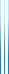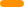# PublicationsPapers

## A tight combinatorial algorithm for submodular maximization subject to a matroid constraint

Yuval Filmus and Justin Ward
FOCS 2012, SICOMP

We present an optimal, combinatorial $1-1/e$ approximation algorithm for monotone submodular optimization over a matroid constraint. Compared to the continuous greedy algorithm due to Calinescu, Chekuri, Pál and Vondrák, our algorithm is extremely simple and requires no rounding. It consists of the greedy algorithm followed by local search. Both phases are run not on the actual objective function, but on a related auxiliary potential function, which is also monotone submodular.

In our previous work on maximum coverage (the preceding paper), the potential function gives more weight to elements covered multiple times. We generalize this approach from coverage functions to arbitrary monotone submodular functions. When the objective function is a coverage function, both definitions of the potential function coincide.

Our approach generalizes to the case where the monotone submodular function has restricted curvature. For any curvature $c$, we adapt our algorithm to produce a $(1-e^{-c})/c$ approximation. This matches results of Vondrák, who has shown that the continuous greedy algorithm produces a $(1-e^{-c})/c$ approximation when the objective function has curvature $c$ with respect to the optimum, and proved that achieving any better approximation ratio is impossible in the value oracle model.

The paper exists in several different versions:

• The conference version only contains the case $c=1$.
• The arXiv version contains the result for general $c$. A similar account can be found in Ward’s thesis.
• The journal version contains a significantly simplified proof of the result for general $c$.
• The extended version includes slightly better approximation ratios for bounded matroid rank, and an improved version of the continuous greedy algorithm.
• The exposition gives a simplified exposition of the main part of the analysis, following ideas of Moran Feldman.

The journal version supersedes the preceding versions.

## BibTeX

@inproceedings{FW2012b,
author = {Yuval Filmus and Justin Ward},
title = {A tight combinatorial algorithm for submodular maximization
subject to a matroid constraint},
booktitle = {53rd Annual {IEEE} Symposium on Foundations of Computer Science ({FOCS} 2012)},
year = {2012},
pages = {659--668}
}

@article{FW2014,
author = {Yuval Filmus and Justin Ward},
title = {Monotone submodular maximization over a matroid via non-oblivious local search},
journal = {SIAM Journal on Computing},
volume = {43},
issue = {2},
year = {2014},
pages = {514--542}
}copy to clipboard